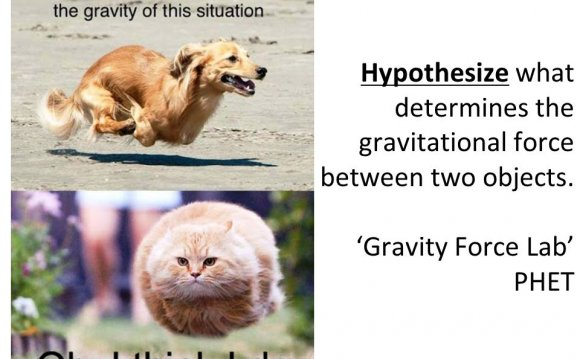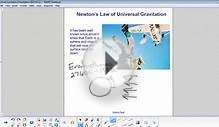# What determines the gravitational force between objects?

July 29, 2023Planet Impact
Teacher Page: Science Background

Index:

How can this be used?

The science background is written by teachers for teachers and students. If you unfamiliar with the information contained in the lesson, this text will give you the background you need to feel confident in using the lesson. Students also can use these pages to do research on related topics or to read as a follow-up to the lesson. The questions marked with an asterisk, *, can be used as higher-level assessment questions.

Science Background:

1. What is gravity?
Gravity is the attraction of every body to every other body due to the masses of each body. The larger the mass, the greater the force. It also depends on the distances: the closer the bodies, the greater the force. Gravity is directed toward the center of a body, and the distance is measured from the center. Gravity keeps the moon going around the earth, the earth going around the sun, and the sun going around the center of the Milky Way.
Gravity is the weakest of the four fundamental forces of nature, yet it is the force that governs motion in the universe. Electromagnetic, weak nuclear, and strong nuclear are the other three forces.

2. What factors determine the force of gravity between two bodies?
The force of gravity (F) depends on the masses of the two bodies (m1, m2), and the distance between the bodies' centers (r). There is a direct proportion between mass and gravitational force: If you double the mass of one body, the gravitational force between them is also doubled. The gravitational force is inversely proportional to the square of the distance: If you double the distance between the two bodies, the force of gravity is reduced to one-fourth its original value. The equation relating these ideas is: F = G(m1m2)/r2 , where G is the universal gravitational constant equal to 6.67 x 10-11 Nm2/kg2 ( or m3/s2kg).

3. What keeps objects in orbit? (What keeps the moon from falling to the earth or the earth from falling into the sun?)
Objects remain in orbit around a massive body due to gravity and their sideways motion. Objects in orbit are moving sideways, approximately at right angles (90 degrees) to the force of gravity. An object would travel in a straight line with a constant speed if it were not for the gravitational attraction of a massive body. The attractive force changes the motion of the object from a straight line to a closed curve, as it begins orbiting the massive body. In effect, the object is "falling" around the massive body

4. What is an unbalanced or net force?
An unbalanced or net force causes changes in an object's speed and/or direction. Only one force acting on a body is unbalanced because there is no counter-force to cancel the force's effects. A person remains at rest when sitting in a chair because there are two balanced forces acting on that person. One of those forces is gravity, which pulls the person downward. The other force is the chair pushing upward on the person. The two forces are equal in magnitude (size) and opposite in direction. So, they balance each other, and the person doesn't change direction or speed. On the other hand, Earth's gravitational influence on the moon is unbalanced. The moon is constantly changing its direction of motion, so it is experiencing acceleration. Any time there is an unbalanced force, the object will undergo a change in direction or speed. So, a change in direction or speed means there is an unbalanced or net force at work.

5. * How does a large body, like a planet, capture a small body, like a comet?
According to Newton's first law of motion, an object in motion tends to remain in straight-line motion at a constant speed unless acted upon by an external, unbalanced or net force. When a comet or asteroid comes close to a body with a large gravitational force, a planet for example, the path of the comet or asteroid is altered due to the unbalanced force of gravity on the body. It moves toward the planet as described in Newton's second law: When an unbalanced force acts on a body, the body experiences acceleration in the direction of the force. A force, which tends to make a body move in a curved path, is called a centripetal force. Occasionally, the comet will be close enough to the planet to become trapped by the gravitational force and will begin orbiting the planet. The comet would like to continue traveling in a straight line, but the planet is pulling the smaller body towards its center, making it travel in a curved path around the planet.

6. Why does the angle of approach affect the ability of a planet to capture a comet?
The more direct the approach, the easier it is for the planet to capture a comet because the comet comes closer to the planet. As discussed above, the closer the objects come to each other, the stronger the force of gravity.

7. What role does speed play in the capture of a comet by a planet?
The faster an object is moving, the greater the kinetic energy. In order for an object to be trapped by the gravity of a planet, the object's kinetic energy (Ek = 1/2 mv2 where Ek = kinetic energy, m = mass of comet and v = speed) must be less than the gravitational potential energy (U = GMm/r where U = potential energy, G = gravitational constant, M = mass of planet, m = mass of comet and r = distance from the center of the planet to the center of the comet). The comet's total energy is equal to the kinetic plus potential energies. But the potential energy is negative, so a comet can only escape a planet's gravitational pull if the kinetic energy is larger than the gravitational potential energy.

8. Does a comet's mass play a role in its capture by a planet? Or, why doesn't a comet's mass affect the path it follows?
When a comet travels near a planet, there is a gravitational force between the comet and the planet (Fg= GMm/r2 where Fg = gravitational force and the others as defined above). This force provides a centripetal acceleration, which changes the comet's path so that it begins orbiting the planet. (Fc= mac where Fc = centripetal force and ac = centripetal acceleration). These two forces are the same. If we set them equal to each other, the mass of the comet factors out of the equation.